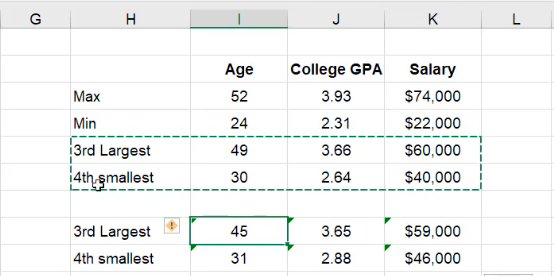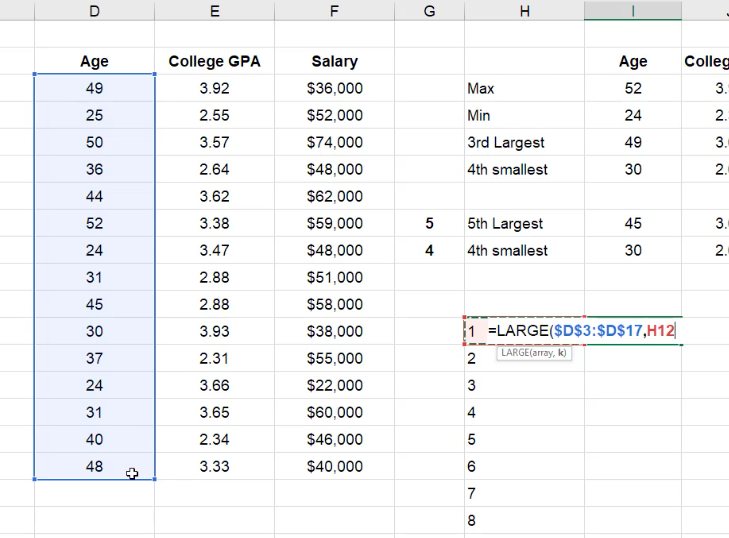# Ranking

In this practice, you will learn how to organize data in an Excel spreadsheet by rank.

Use this workbook for the chapter.

## Maximum and Minimum

Looking at the table, notice the different columns: employee, position, age, college GPA, and salary. Let's suppose that we wanted to find the oldest age in this list of employees.

We could scan the list with our eyes and find the answer that way, but suppose the list was much longer, perhaps thousands of rows. You wouldn’t be able to do that. However, there is a way to have Excel calculate the answer for us.

In this practice, we will explore how to find the maximum age, the minimum, the third-largest, and the fourth smallest. Type these into 4 cells in the same column, then copy and paste the headers for Age, College GPA, and Salary in the next three columns. (See Figure 7.1)

Let’s begin with the maximum age. Complete the following steps.

1. Type =MAX into the cell, followed by an open parentheses.
2. Select all the values in the Age column, then close the parentheses and push Enter.

This will automatically calculate the maximum age from the selected range. Your formula should look similar to the one in Figure 7.2.

To calculate the minimum age, do the following in the cell beside the one labeled Min.

1. Type =MIN into the cell, followed by an open parentheses.
2. Select all the values in the same range as before, then close the parentheses and push Enter

This will automatically calculate the minimum age from the selected range.

## Large and Small Functions

To find the third-largest, there is a formula called “large” that allows you to find within a given array, what largest number you would find. This is represented in the formula as k. This variable tells Excel which number “largest” you’d like to identify. For example, in the circumstance of third largest, k would equal three. (See Figure 7.3)

To calculate the third-largest, do the following in the cell beside the one labeled Min.

1. Type =LARGE into the cell, followed by an open parentheses.
2. Select all the values in the same range as before, replace the spot for k with 3, then close the parentheses and push Enter.

This principle is also applicable to finding, for example, the fourth smallest. This formula is called “small.”

1. Type =SMALL into the cell, followed by an open parentheses.
2. Select all the values in the same range as before, replace the spot for k with 4, then close the parentheses and push Enter. (See Figure 7.4)

## Matching the Format

Now, because we didn't anchor the references for these functions, we can drag them across to calculate the maximum and the minimum for GPA and salary as well. Since we want the number formats to match, use the format painter.

1. Select one row of the original age, GPA, and salary.

1. This will automatically adjust the format to match that of the original reference. (See Figure 7.7)

## Cell Referencing

One slight alteration we can do to our formulas to make them even more dynamic is that if we didn’t want to be “hard-coding” or just typing in, for example, a three for “third largest” we can reference an additional cell.

1. Copy the cells with the =LARGE and =SMALL functions, and paste them below. (See Figure 7.8)Figure 7.8

2. Notice that the pasted cells are now referencing the wrong set of cells.
3. Delete the incorrect information.
4. Off to the side, type a beside the 3rd Largest cell, and a beside the 4th Smallest cell.
5. Type =LARGE in the cell beside the 3rd Largest, choose your array, (which is just all of the ages,) and then reference the cell. (See Figure 7.9)
6. Hit F4 to anchor the formula so that as you drag and copy it over, it won't move.
7. Repeat with the =SMALL function.
8. Drag over to the right, hover over the icon in the corner, and choose Fill Without Formatting to maintain the number formats it had previously.
1. Now, if instead of the third-largest we'd like to see, for example, the fifth-largest, we can change the to a 5, and the referenced information will change along with it.
2. Manually change the label to match, from third largest to fifth largest.

## Numbered List

We can also create a numbered list to rank the items from smallest to largest.

1. You can manually type and drag a list of, for example, 1–8, or you can use the fill series option as learned in a previous video.
2. Then, type =LARGE, select the array, and then anchor it, or make it an absolute reference, by pressing F4
3. Then, for k, (which rank we want), we want the first largest, so you will select the number 1 from the list you previously created. (See Figure 7.10)Figure 7.10

4. You can then drag it down, and because you have attached it to the proper references, it should fill in with the corresponding numbers.
5. Label the list with the word Large.

You’ll notice that if you attempt to drag over the ages for the GPA and salary, it will give you an error. This has to do with the anchoring because rather than being referenced to the number 1, k is now referenced to 52

To anchor just the cell, you will need to anchor to just the row, rather than the column and the row.

1. Change your formula so that only the row is being referenced. (See Figures 7.11 and 7.12)
1. This makes it so that it won’t move when you move cells down, but it will move columns to the right if dragged over.
2. Use the format painter to keep the data looking consistent.

You could do the same exercise with the small formula.

## Rank Function

For our next example of ranking, let’s say that instead of creating a list off to the side, we want to add a column into our data to give it a rank relative to the list and will rank every single item in the list.

1. Select columns G through L, right-click, and select Insert.
1. This will shift all the formulas to the right so you don’t lose them but have a space to work in.
2. Label columns for ranking each of the elements.
1. The ampersand gives you the ability to combine terms.
1. Type =”Rank ”&
2. Select D2, the cell labeled Age
3. Press Enter.
4. Drag this cell over, and the information should fill in until all the columns are labeled properly.

## RANK.AVG

If there's more than one age in the list that are the same and you choose RANK.AVG, the rank assigned to both of them will be the average of their rank.

1. Select the first cell under Rank Age, G3, and type =RANK.AVG.
2. Select the corresponding Age cell in the same row, D3, and type a comma after.
3. Select the entire Age array, and anchor just the rows.
4. Type a comma, and it will give you the option between descending or ascending. Choose descending (the largest number gets the highest rank).
5. Note that when the formula is complete, it will rank the age with a dollar sign. Update the format by selecting Number rather than Currency. Remove the decimals.
6. Drag this cell all the way down.
7. Drag that new array across.
1. If your anchoring is set up correctly, each cell should correlate with the number in the same row in the assigned column of that information.
2. If you expand the decimals, note that some ranks contain a .5 at the end. This is because the RANK.AVG formula is being used. It is averaging the two places to give you together and giving them the average of the two ranks.
1. For example, the average of place 10 and place 11 would be 10.5.

## RANK.EQ

If you choose rank.eq, instead of averaging the two ranks that happen to be the same, it will take the highest of the two and it will assign that rank to both of them.

1. Copy the formulas from the rank titles by referencing them.
2. Add a note in the column above that says AVG for the averaged ranks, and EQ for the ones you are about to complete.
1. Color code them using the fill colors.
3. Set up the rank formula again, but this time, choose RANK.EQ instead of RANK.AVG.
1. Don’t forget to anchor only the rows and not the columns.
4. Copy the cell over to the rest of the columns.
5. Copy these three cells down to the rest of the rows.
6. Change the formatting from Currency to Numbers.
1. Notice that there’s no .5 in the decimal places this time because the higher rank of the two that match is being used.
2. For example, if two values at the 10 and 11 place were the same, they would both become 10.

That’s how the RANK function works, along with the MIN, MAX, LARGE, and SMALL functions.